Author: Oscar Cronquist Article last updated on May 04, 2022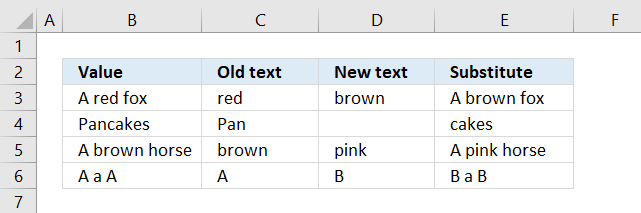Formula in cell E3:

=SUBSTITUTE(B3,C3,D3)

The SUBSTITUTE function replaces a specific text string in a value. It is case sensitive meaning it differentiates between upper and lower letters.

The image above shows the SUBSTITUTE function cell E3 substituting the word "red" with "brown" based on value in cell B3.

## 1. SUBSTITUTE Function Syntax

SUBSTITUTE(text, old_text, new_text, [instance_num])

## 2. SUBSTITUTE Function Arguments

 text Required. The value you want to substitute text in. old_text Required. The text string you are looking for in the value. new_text Required. The text you want to substitute. [instance_num] Optional. Which instance to replace. If not entered every instance is substituted.

## 3. SUBSTITUTE function example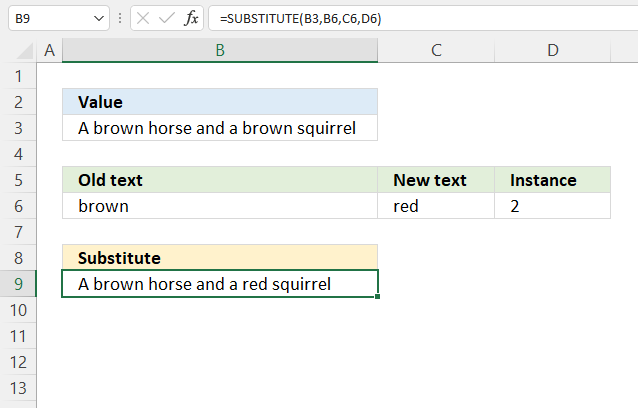The SUBSTITUTE function, in the above example, substitutes the word "brown" with "red" at the second found instance. Cell B6 contains "brown", cell C6 contains "red" and cell D6 contains 2 representing which instance to replace.

Formula in cell B9:

=SUBSTITUTE(B3,B6,C6,D6)

## 4. SUBSTITUTE function wildcard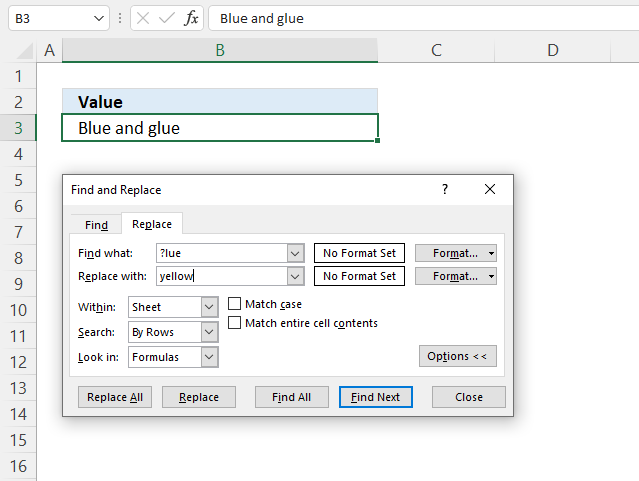You can't use wildcard characters in the SUBSTITUTE function, however, you can use the built-in feature "Find and Replace" to do the same thing.

You can use the following characters:

• ? (question mark) - a single character
• * (asterisk) - any number of characters zero included

Here are the steps to use the "Find and Replace" dialog box:

1. Select a cell range you want to use.
2. Press CTRL + H to open the "Find and Replace" dialog box.
3. Type the search string in " Find what:".
4. Type the substitution string in "Replace with:".
5. Press with the left mouse button on the "Replace" button to replace strings in a single cell.
Press with the left mouse button on the "Replace All" button to replace strings in all cells on the active worksheet.
Tip! Press with left mouse button on tab "Find" to first search the cells without replacing strings to make sure the right cells and strings get changed.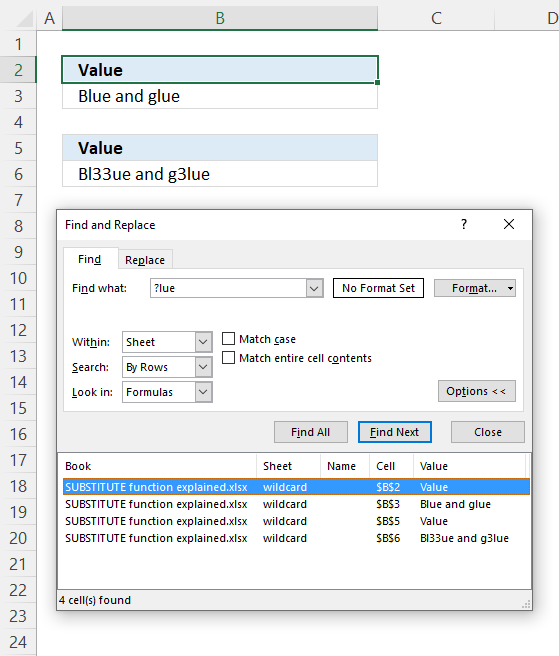## 5. How to substitute quotation marks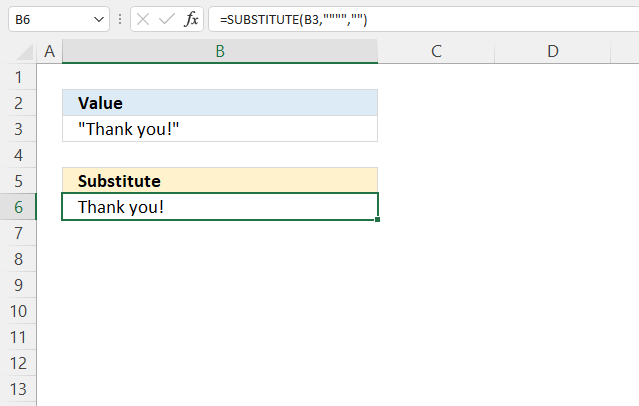The image above demonstrates how to remove quotation marks using the SUBSTITUTE function.

Formula in cell B6:

=SUBSTITUTE(B3,"""","")

## 6. How to substitute multiple strings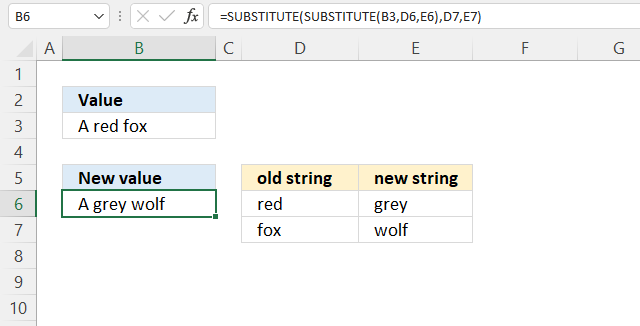You can nest the SUBSTITUTE function to substitute multiple values in one calculation, however, the formula grows quickly if you have many strings to substitute.

Formula in cell B6:

=SUBSTITUTE(SUBSTITUTE(B3, D6, E6), D7, E7)

### Explaining formula

#### Step 1 - Substitute first string

SUBSTITUTE(B3, D6, E6)

becomes

SUBSTITUTE("A red fox", "red", "grey")

and returns "A grey fox".

#### Step 2 - Substitute second string

SUBSTITUTE(SUBSTITUTE(B3, D6, E6), D7, E7)

becomes

SUBSTITUTE("A grey fox", D7, E7)

becomes

SUBSTITUTE("A grey fox", "fox", "wolf")

and returns "A grey wolf".

Substitute multiple text strings [UDF]

## 7. SUBSTITUTE function from right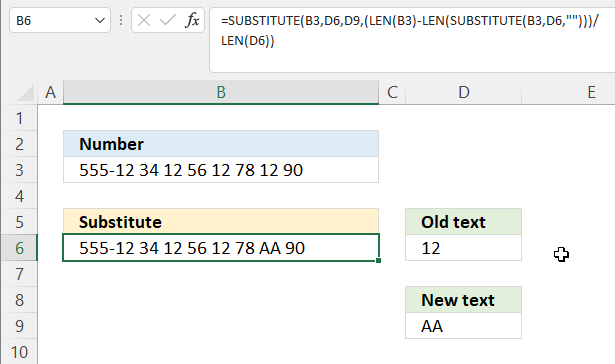Formula in cell B6:

=SUBSTITUTE(B3, D6, D9, (LEN(B3)-LEN(SUBSTITUTE(B3, D6, "")))/LEN(D6))

### Explaining formula

#### Step 1 - Substitute string with nothing

SUBSTITUTE(B3, D6, "")

becomes

SUBSTITUTE("555-12 34 12 56 12 78 12 90",12,"")

and returns

"555- 34 56 78 90".

#### Step 2 - Count characters

The LEN function counts the number of characters.

LEN(value)

LEN(SUBSTITUTE(B3, D6, ""))

becomes

LEN("555- 34 56 78 90")

and returns 19. "555- 34 56 78 90" contains 19 characters.

#### Step 3 - Count characters in cell B3

LEN(B3)

becomes

LEN("555-12 34 12 56 12 78 12 90")

and returns 27.

#### Step 4 - Subtract character counts

LEN(B3)-LEN(SUBSTITUTE(B3, D6, ""))

becomes

27 - 19 equals 8.

#### Step 5 - Divide with old text character count

The division character lets you divide numbers in an Excel formula. The parentheses allow you to control the order of calculation.

(LEN(B3)-LEN(SUBSTITUTE(B3, D6, "")))/LEN(D6)

becomes

(27 - 18)/LEN(12)

becomes

(27 - 18)/2

becomes

8/2 equals 4. There are four instances of value 12 in cell B3. "555-12 34 12 56 12 78 12 90"

#### Step 6 - Substitute value based on instance

SUBSTITUTE(B3, D6, D9, (LEN(B3)-LEN(SUBSTITUTE(B3, D6, "")))/LEN(D6))

becomes

SUBSTITUTE("555-12 34 12 56 12 78 12 90", 12, "AA", 4)

and returns "555-12 34 12 56 12 78 AA 90".

## 8. Substitute all letters with a given string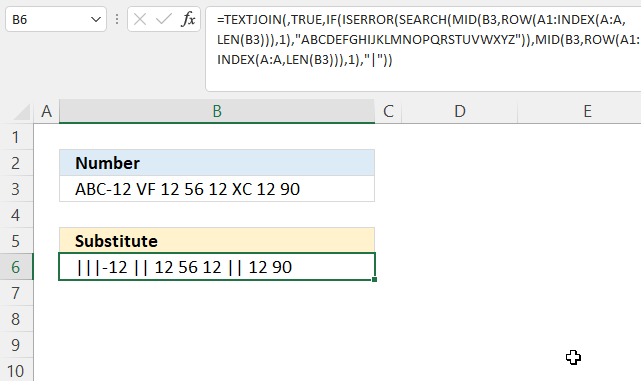This formula substitutes all letters, upper and lower letters, to a given string.

Array formula in cell B6:

=TEXTJOIN(, TRUE, IF(ISERROR(SEARCH(MID(B3, ROW(A1:INDEX(A:A, LEN(B3))), 1), "ABCDEFGHIJKLMNOPQRSTUVWXYZ")), MID(B3, ROW(A1:INDEX(A:A, LEN(B3))), 1), "|"))

The last part of the formula contains the string you want to use, bolded in the formula below.

TEXTJOIN(, TRUE, IF(ISERROR(SEARCH(MID(B3, ROW(A1:INDEX(A:A, LEN(B3))), 1), "ABCDEFGHIJKLMNOPQRSTUVWXYZ")), MID(B3, ROW(A1:INDEX(A:A, LEN(B3))), 1), "|"))

### 8.1 How to enter an array formula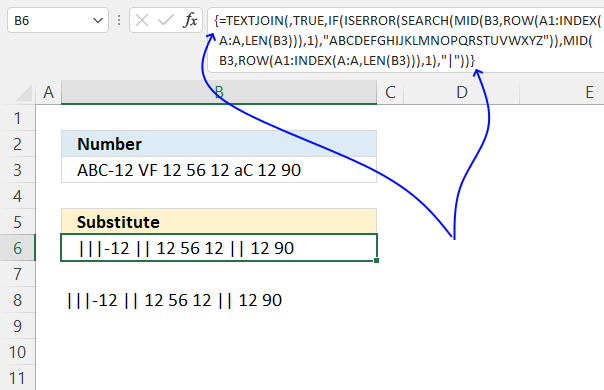Excel 365 users may enter the formula as a regular formula, no need to follow the steps below.

1. Copy above formula
2. Double press with the left mouse button on cell B3, a prompt appears.
3. Paste the formula.
4. Press and hold CTRL + SHIFT simultaneously.
5. Press Enter once.
6. Release all keys.

The formula bar contains a leading and trailing curly bracket if you followed the above steps, it indicates that the formula is an array formula. Don't enter these characters yourself.

### 8.2 Explaining formula

#### Step 1 - Count characters

The LEN function counts the number of characters.

LEN(value)

LEN(B3)

becomes

LEN("ABC-12 VF 12 56 12 aC 12 90")

and returns 27.

#### Step 2 - Create a cell ref

The INDEX function lets you build a dynamic cell reference.

INDEX(A:A, LEN(B3))

becomes

INDEX(A:A, 27)

and returns A27.

#### Step 3 - Create a cell ref to a cell range

The colon character lets you append a cell ref to a cell ref.

A1:INDEX(A:A, LEN(B3))

returns A1:A27.

#### Step 4 - Create row numbers

The ROW function calculates the row number of a cell reference.

ROW(ref)

ROW(A1:INDEX(A:A, LEN(B3)))

becomes

ROW(A1:A27)

and returns {1; 2; 3; 4; 5; 6; 7; 8; 9; 10; 11; 12; 13; 14; 15; 16; 17; 18; 19; 20; 21; 22; 23; 24; 25; 26; 27}.

#### Step 5 - Create an array containing each character in cell B3

The MID function returns a substring from a string based on the starting position and the number of characters you want to extract.

MID(B3, ROW(A1:INDEX(A:A, LEN(B3))), 1)

becomes

MID("ABC-12 VF 12 56 12 aC 12 90", {1; 2; 3; 4; 5; 6; 7; 8; 9; 10; 11; 12; 13; 14; 15; 16; 17; 18; 19; 20; 21; 22; 23; 24; 25; 26; 27}, 1)

and returns

{"A"; "B"; "C"; "-"; "1"; "2"; " "; "V"; "F"; " "; "1"; "2"; " "; "5"; "6"; " "; "1"; "2"; " "; "a"; "C"; " "; "1"; "2"; " "; "9"; "0"}.

#### Step 6 - Calculate position of each character in the alphabet

The SEARCH function returns a number representing the position of character at which a specific text string is found reading left to right. It is not a case-sensitive search.

SEARCH(find_text,within_text, [start_num])

SEARCH(MID(B3, ROW(A1:INDEX(A:A, LEN(B3))), 1), "ABCDEFGHIJKLMNOPQRSTUVWXYZ")

becomes

SEARCH({"A"; "B"; "C"; "-"; "1"; "2"; " "; "V"; "F"; " "; "1"; "2"; " "; "5"; "6"; " "; "1"; "2"; " "; "a"; "C"; " "; "1"; "2"; " "; "9"; "0"}, "ABCDEFGHIJKLMNOPQRSTUVWXYZ")

and returns

{1; 2; 3; #VALUE!; #VALUE!; #VALUE!; #VALUE!; 22; 6; #VALUE!; #VALUE!; #VALUE!; #VALUE!; #VALUE!; #VALUE!; #VALUE!; #VALUE!; #VALUE!; #VALUE!; 1; 3; #VALUE!; #VALUE!; #VALUE!; #VALUE!; #VALUE!; #VALUE!}

Note that the SEARCH function returns an error value if the character is not found in the alhpabet.

#### Step 7 - Find error values in the array

The ISERROR function returns TRUE if a value is an error value.

ISERROR(value)

ISERROR(SEARCH(MID(B3, ROW(A1:INDEX(A:A, LEN(B3))), 1), "ABCDEFGHIJKLMNOPQRSTUVWXYZ"))

becomes

ISERROR({1; 2; 3; #VALUE!; #VALUE!; #VALUE!; #VALUE!; 22; 6; #VALUE!; #VALUE!; #VALUE!; #VALUE!; #VALUE!; #VALUE!; #VALUE!; #VALUE!; #VALUE!; #VALUE!; 1; 3; #VALUE!; #VALUE!; #VALUE!; #VALUE!; #VALUE!; #VALUE!})

and returns

{FALSE; FALSE; FALSE; TRUE; TRUE; TRUE; TRUE; FALSE; FALSE; TRUE; TRUE; TRUE; TRUE; TRUE; TRUE; TRUE; TRUE; TRUE; TRUE; FALSE; FALSE; TRUE; TRUE; TRUE; TRUE; TRUE; TRUE}.

#### Step 8 - Replace TRUE with the corresponding character

The Boolean value FALSE will be replaced with a "|" character.

IF(ISERROR(SEARCH(MID(B3, ROW(A1:INDEX(A:A, LEN(B3))), 1), "ABCDEFGHIJKLMNOPQRSTUVWXYZ")), MID(B3, ROW(A1:INDEX(A:A, LEN(B3))), 1), "|")

becomes

IF({FALSE; FALSE; FALSE; TRUE; TRUE; TRUE; TRUE; FALSE; FALSE; TRUE; TRUE; TRUE; TRUE; TRUE; TRUE; TRUE; TRUE; TRUE; TRUE; FALSE; FALSE; TRUE; TRUE; TRUE; TRUE; TRUE; TRUE}, MID(B3, ROW(A1:INDEX(A:A, LEN(B3))), 1), "|")

becomes

IF({FALSE; FALSE; FALSE; TRUE; TRUE; TRUE; TRUE; FALSE; FALSE; TRUE; TRUE; TRUE; TRUE; TRUE; TRUE; TRUE; TRUE; TRUE; TRUE; FALSE; FALSE; TRUE; TRUE; TRUE; TRUE; TRUE; TRUE}, {"A"; "B"; "C"; "-"; "1"; "2"; " "; "V"; "F"; " "; "1"; "2"; " "; "5"; "6"; " "; "1"; "2"; " "; "a"; "C"; " "; "1"; "2"; " "; "9"; "0"}, "|")

and returns

{"|";"|";"|";"-";"1";"2";" ";"|";"|";" ";"1";"2";" ";"5";"6";" ";"1";"2";" ";"|";"|";" ";"1";"2";" ";"9";"0"}.

#### Step 9 - Concatenate characters

The TEXTJOIN function concatenates cell values.

TEXTJOIN(delimiterignore_emptytext1[text2], ...)

TEXTJOIN(, TRUE, IF(ISERROR(SEARCH(MID(B3, ROW(A1:INDEX(A:A, LEN(B3))), 1), "ABCDEFGHIJKLMNOPQRSTUVWXYZ")), MID(B3, ROW(A1:INDEX(A:A, LEN(B3))), 1), "|"))

becomes

TEXTJOIN(, TRUE, {"|";"|";"|";"-";"1";"2";" ";"|";"|";" ";"1";"2";" ";"5";"6";" ";"1";"2";" ";"|";"|";" ";"1";"2";" ";"9";"0"})

and returns "|||-12 || 12 56 12 || 12 90".

Excel 365 dynamic array formula in cell B6:

=LET(x,MID(B3,ROW(A1:INDEX(A:A,LEN(B3))),1),TEXTJOIN(,TRUE,IF(ISERROR(SEARCH(x,"ABCDEFGHIJKLMNOPQRSTUVWXYZ")),x,"|")))

## 9. Substitute all numbers with a given character/string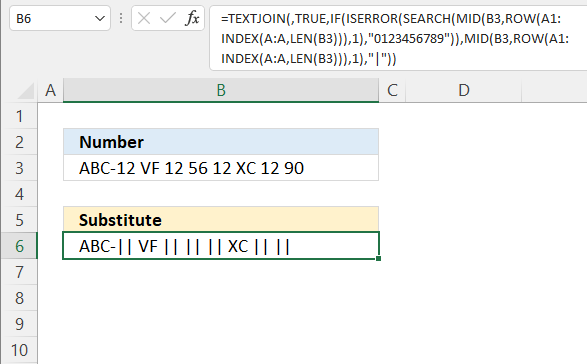Array formula in cell

=TEXTJOIN(,TRUE,IF(ISERROR(SEARCH(MID(B3,ROW(A1:INDEX(A:A,LEN(B3))),1),"0123456789")),MID(B3,ROW(A1:INDEX(A:A,LEN(B3))),1),"|"))

How to enter an array formula

Read section 8 for an formula explanation.

## 10. Substitute all characters except numbers and letters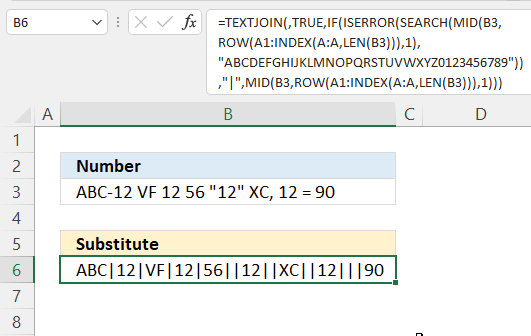The image above demonstrates an array formula that replaces all characters except upper and lower letters and digits to a given string, in this example |.

Array formula in cell B6:

=TEXTJOIN(, TRUE, IF(ISERROR(SEARCH(MID(B3, ROW(A1:INDEX(A:A, LEN(B3))), 1), "ABCDEFGHIJKLMNOPQRSTUVWXYZ0123456789")), "|", MID(B3, ROW(A1:INDEX(A:A, LEN(B3))), 1)))

How to enter an array formula

Read section 8 for a formula explanation.

## 11. SUBSTITUTE function based on table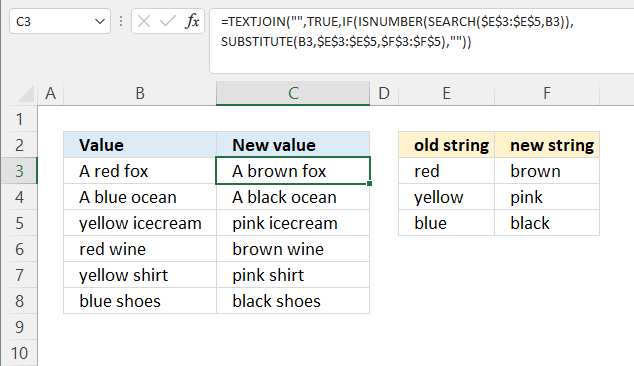The formula in cell C3 substitutes the value in cell B3 using the table in cells E2:F5. This formula works if only one string is found, it returns a blank if nothing is found.

Array formula in cell C3:

=TEXTJOIN("", TRUE, IF(ISNUMBER(SEARCH(\$E\$3:\$E\$5, B3)), SUBSTITUTE(B3, \$E\$3:\$E\$5, \$F\$3:\$F\$5), ""))

How to enter an array formula

### Explaining formula

#### Step 1 - Search for each old string in the table simultaneously

The SEARCH function returns a number representing the position of character at which a specific text string is found reading left to right. It is not a case-sensitive search.

SEARCH(find_text,within_text, [start_num])

SEARCH(\$E\$3:\$E\$5, B3)

becomes

SEARCH({"red";"yellow";"blue"},"A red fox")

and returns {3; #VALUE!; #VALUE!}. This means that the first value "red" in the array is found at character position 3 in "A red fox".

#### Step 2 - Find errors

The ISNUMBER function returns TRUE if a value is a number.

ISNUMBER(value)

ISNUMBER(SEARCH(\$E\$3:\$E\$5, B3))

becomes

ISNUMBER({3; #VALUE!; #VALUE!})

and returns {TRUE; FALSE; FALSE}.

#### Step 3 - Substitute strings

SUBSTITUTE(B3, \$E\$3:\$E\$5, \$F\$3:\$F\$5)

becomes

SUBSTITUTE("A red fox",{"red"; "yellow"; "blue"},{"brown"; "pink"; "black"})

and returns

{"A brown fox"; "A red fox"; "A red fox"}.

#### Step 4 - Replace TRUE with the corresponding value

The IF function returns one value if the logical test is TRUE and another value if the logical test is FALSE.

IF(logical_test, [value_if_true], [value_if_false])

IF(ISNUMBER(SEARCH(\$E\$3:\$E\$5, B3)), SUBSTITUTE(B3, \$E\$3:\$E\$5, \$F\$3:\$F\$5), "")

becomes

IF({TRUE; FALSE; FALSE}, {"A brown fox"; "A red fox"; "A red fox"}, "")

and returns

{"A brown fox"; ""; ""}.

#### Step 5 - Concatenate strings

The TEXTJOIN function concatenates cell values.

TEXTJOIN(delimiterignore_emptytext1[text2], ...)

TEXTJOIN("", TRUE, IF(ISNUMBER(SEARCH(\$E\$3:\$E\$5, B3)), SUBSTITUTE(B3, \$E\$3:\$E\$5, \$F\$3:\$F\$5), ""))

becomes

TEXTJOIN("", TRUE, {"A brown fox"; ""; ""})

and returns "A brown fox".